Graphing

Graphing

 Site: TBAISD Moodle Course: Michigan Algebra I Preview 2012 Book: Graphing Printed by: Guest user Date: Saturday, August 8, 2020, 05:26 AM

Introduction

There are numerous ways to solve quadratic equations. This unit will cover graphing, using square roots, factoring, the quadratic formula, and completing the square.

When solving a quadratic equation, there will be two, one or no real solutions. When there are no real solutions the solutions can be represented by the imaginary number i. Since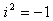, the complex number i is defined as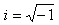. This number can help to define any square root of a negative number.

Example 1

Simplify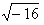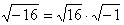, the square roots can be simplified to 4i.

Example 2

Simplify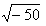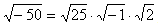, the square roots can be simplified to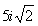.

Listen

Solutions

In general, graphing provides a good way of approximating solutions to quadratic equations as long as the graph has x-intercepts. When a quadratic equation is written in the form ax2 + bx + c = 0, the solutions will be the value(s) of x when y = 0. Another name for these solutions are x-intercepts or roots. A graphing calculator can be used to estimate these solutions. Remember, quadratic equations can have two, one, or no real solutions. Therefore, the graphs will have two, one, or no x -intercepts.

Listen

Example

Find the solutions to the equation 2x2 + 4x - 3 = 0 by graphing.

Step 1. Rewrite the equation by substituting a y in the place of the zero.

y = 2x2 + 4x - 3

Step 2. Use a graphing calculator to make a graph of the function.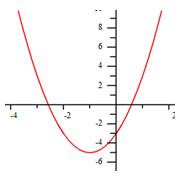Step 3. Does the graph have two, one or no x-intercepts (solutions)?

This graph has 2 x-intercepts, therefore look for 2 solutions to the equation.

Step 4. Use the calculate zeros command to calculate the solutions (x-intercepts) of the equation.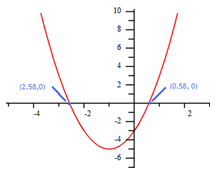*Note: Sometimes the solutions will appear on the table. Use the table function of a graphing calculator and find the values on the table where the y -coordinate is zero.

Video Lesson

To learn how to solve quadratic equations by graphing, select the following link:

Guided Practice

To solidify your understanding of solving quadratics by graphing, visit the following link to Holt, Rinehart and Winston Homework Help Online. It provides examples, video tutorials and interactive practice with answers available. The Practice and Problem Solving section has two parts. The first part offers practice with a complete video explanation for the type of problem with just a click of the video icon. The second part offers practice with the solution for each problem only a click of the light bulb away.

Practice

Solve by Graphing Worksheet